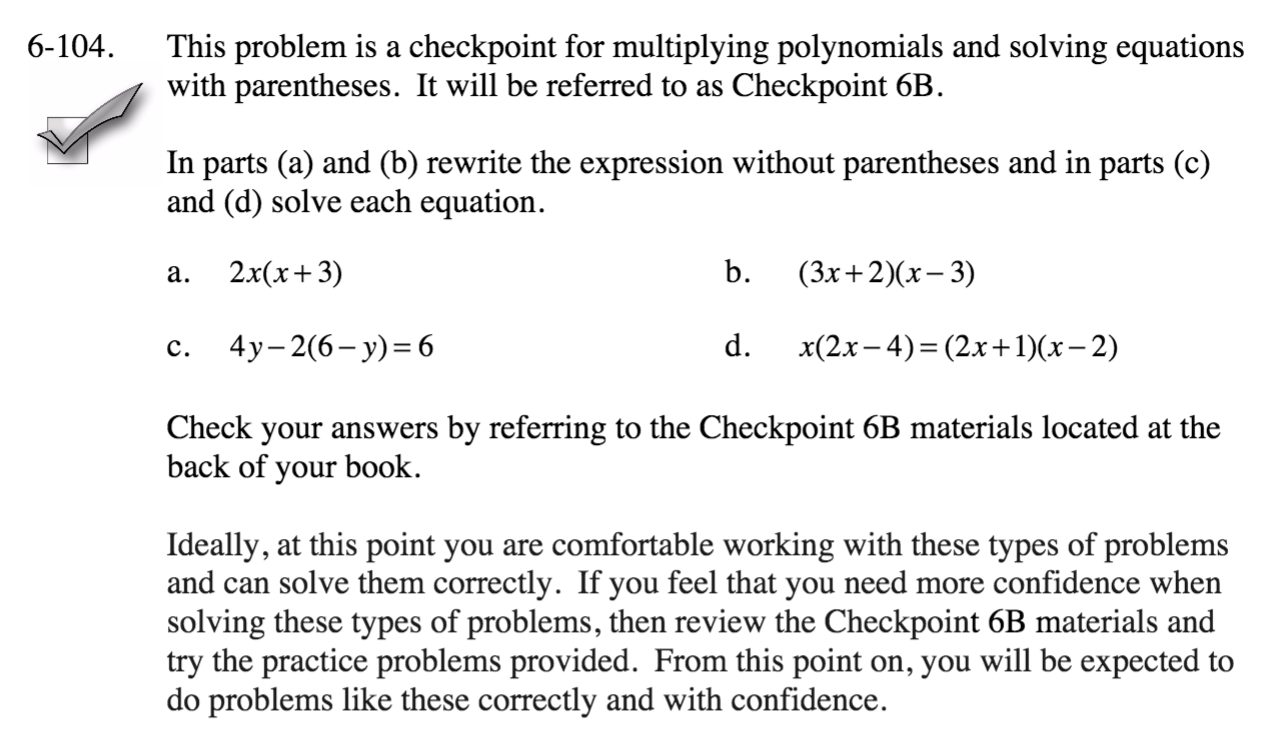### Home > CCA > Chapter 6 > Lesson 6.2.4 > Problem6-104

6-104.
1. This problem is a checkpoint for multiplying polynomials and solving equations with parentheses. It will be referred to as Checkpoint 6B.

2.In parts (a) and (b) rewrite the expression without parentheses and in parts (c) and (d) solve each equation. Homework Help ✎

1. 2x(x + 3)

2. (3x + 2)(x − 3)

3. 4y – 2(6 − y) = 6

4. x(2x − 4) = (2x + 1)(x − 2)

3. Check your answers by referring to the Checkpoint 6B materials.

4. Ideally, at this point you are comfortable working with these types of problems and can solve them correctly. If you feel that you need more confidence when solving these types of problems, then review the Checkpoint 6B materials and try the practice problems provided. From this point on, you will be expected to do problems like these correctly and with confidence.Answers and extra practice are located in the back of your printed textbook or in the Reference Tab of your eBook.
If you have an eBook for CCA, login and then click the following link: Checkpoint 6B: Multiplying Polynomials and Solving Equations with Parentheses